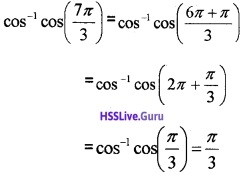# Plus Two Maths Chapter Wise Questions and Answers Chapter 2 Inverse Trigonometric Functions

Students can Download Chapter 2 Inverse Trigonometric Functions Questions and Answers, Plus Two Maths Chapter Wise Questions and Answers helps you to revise the complete Kerala State Syllabus and score more marks in your examinations.

## Kerala Plus Two Maths Chapter Wise Questions and Answers Chapter 2 Inverse Trigonometric Functions

### Plus Two Maths Inverse Trigonometric Functions Three Mark Questions and Answers

Question 1.
Prove the following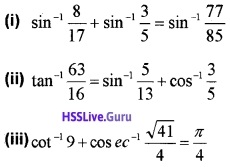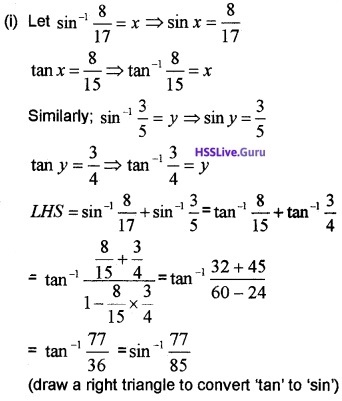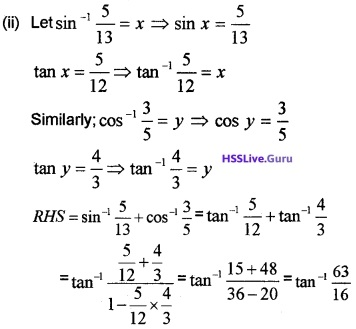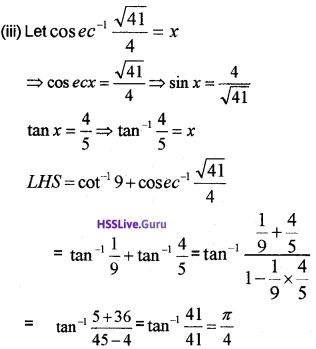Question 2.
Find the value of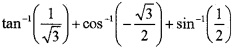Question 3.
If tan-1x + tan-1y + tan-1z = π, show that x + y + z = xyz
Given;
tan-1x + tan-1y + tan-1z = π
⇒ tan-1x + tan-1y = π – tan-1z⇒ x + y + z = xyz.Question 4.
Match the followingQuestion 5.
Solve 2 tan-1(cos x) = tan-1(2 cos x)
2 tan-1(cosx) = tan-1(2cosx)
⇒ $$\frac{2 \cos x}{1-\cos ^{2} x}$$ = 2cosx
⇒ 1 = 1 – cos2 x ⇒ 1 = sin2x
⇒ x = ±$$\frac{\pi}{2}$$.Question 6.
Solve the following

1. 2tan-1(cosx) = tan-1(2cosecx)
2. tan-12x + tan-13x = $$\frac{\pi}{4}$$

1. 2tan-1(cosx) = tan-1(2cosecx)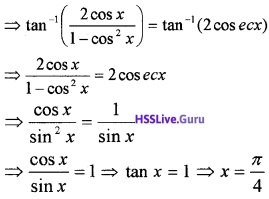2. tan-12x + tan-13x = $$\frac{\pi}{4}$$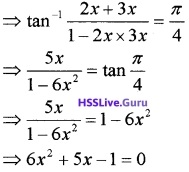⇒ (6x – 1)(x + 1) = 0
⇒ x = $$\frac{1}{6}$$, x = – 1
Since x = – 1 does not satisfy the equation, as the LHS becomes negative. So x = $$\frac{1}{6}$$.Question 7.
Solve 2 tan-1(cos x) = tan-1(2 cos x)
2 tan-1(cos x) = tan-1(2 cos x)
⇒ $$\frac{2 \cos x}{1-\cos ^{2} x}$$ = 2cosx
⇒ 1 = 1 – cos2 x
⇒ 1 = sin2 x ⇒ x = ±$$\frac{\pi}{2}$$

### Plus Two Maths Inverse Trigonometric Functions Four Mark Questions and Answers

Question 1.
Prove that $$\sin ^{-1} \frac{12}{13}+\cos ^{-1} \frac{4}{5}+\tan ^{-1} \frac{63}{16}=\pi$$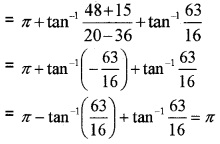Question 2.

1. Find the principal value of sec-1$$\left(-\frac{2}{\sqrt{3}}\right)$$ (1)
2. if sin$$\left(\sin ^{-1}\left(\frac{1}{5}\right)+\cos ^{-1}(x)\right)=1$$, then find the value of x. (3)

1. principal value of:2. find the value of x:Question 3.
Solve the followingThe value x = –$$\frac{\sqrt{3}}{\sqrt{28}}$$ makes the LHS negative, so rejected.Question 4.
(i) Choose the correct answer from the bracket. cos(tan-1 x), |x| < 1 is equal to (1)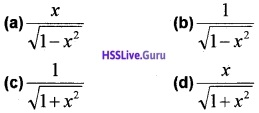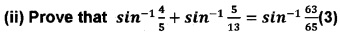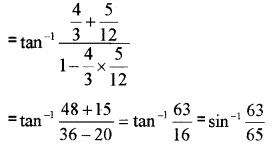(draw a right triangle to convert ‘tan’ to ‘sin’).Question 5.
(i) In which quadrants are the graph of cos-1 (x) lies, x ∈ [-1,1 ] (1)
(ii) If cos-1x + cos-1y = $$\frac{\pi}{3}$$, then
sin-1x + sin-1y = ……… (3)
(a) $$\frac{2 \pi}{3}$$
(b) $$\frac{\pi}{3}$$
(c) $$\frac{\pi}{6}$$
(d) $$\frac{\pi}$$
(iii) If tan-1x + tan-1y = $$\frac{\pi}{4}$$ then prove that x + y + xy = 1 (2)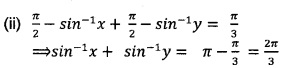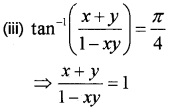⇒ x + y = 1 – xy ⇒ x + y + xy = 1.Question 6.
(i) sin(tan-1(1)) is equal to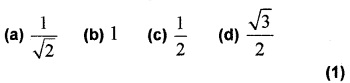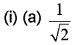### Plus Two Maths Inverse Trigonometric Functions Six Mark Questions and Answers

Question 1.
Show that sin-1$$\frac{3}{5}$$ – sin-1$$\frac{8}{17}$$ = cos-1$$\frac{84}{85}$$.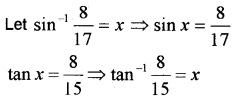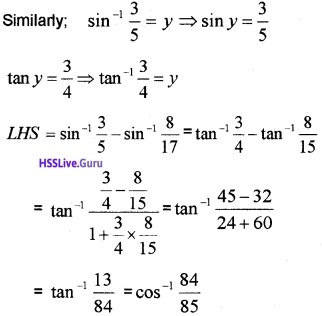(draw a right triangle to convert ‘tan’ to ‘cos’).Question 2.
(i) Choose the correct answer from the Bracket.
If cos-1x = y, then y is equal to (1)
(a) π ≤ y ≤ π
(b) 0 ≤ y ≤ π
(c) $$-\frac{\pi}{2}$$ ≤ y ≤ $$\frac{\pi}{2}$$
(d) 0 ≤ y ≤ π
(ii) Find the value of cos-1 cos$$\left(\frac{7 \pi}{3}\right)$$ (3)
(iii) Solve for x if, tan-1$$\left(\frac{1+x}{1-x}\right)$$ = 2 tan-1x (2)
(ii) Here $$\left(\frac{7 \pi}{3}\right)$$ lie outside the interval [0, π]. TO make it in the interval proceed as follows.